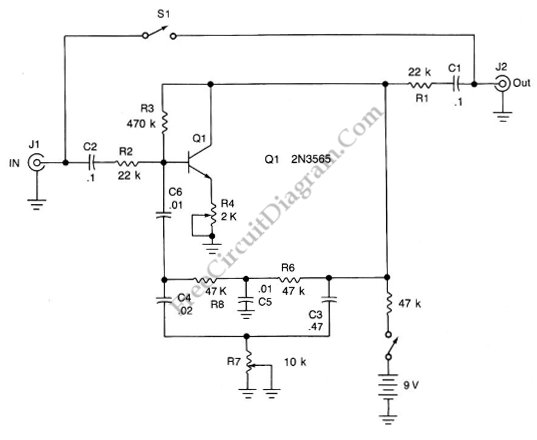## Phase-Sensitive Sinusoidal Signals Detector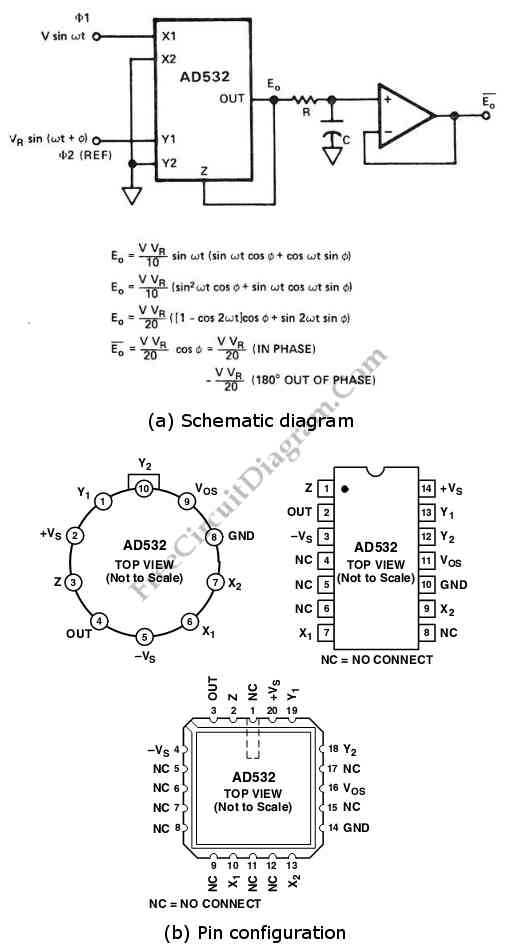Using the phase sensitive detector circuit below the magnitude of sinusoidal signal can be measured. Beside that, this circuit also can be used to measures 180°-out-of-phase inputs with proper polarity. But, it depends on the relationship to the reference with less than 1% error. This circuit uses a AD741J op amp. Here is the schematic diagram of the circuit and […]

## Single Chip Divider Circuit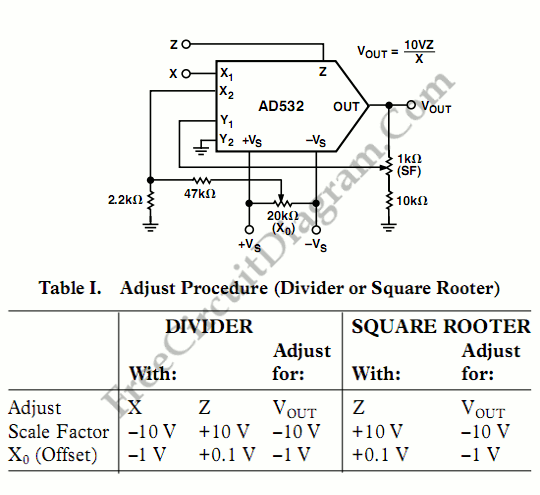We can build a divider circuit using only a single chip component, a special purpose integrated circuit AD532. We can configure the AD532 as a two quadrant divider by connecting the multiplier cell in the feedback loop of the op amp and using the Z terminal as a signal input, as shown in the following figure. For your note, the […]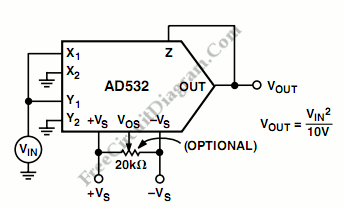The following schematic diagram shows a squaring circuit.  Basically, this circuit is a simple variation of  multiplier circuit. However, the differential input capability of the AD532 can be used to obtain a positive or negative output response to the input. This circuit has useful feature which is suitable for control applications, as it might eliminate the need for an additional […]

## Single Chip Circuit for Multiplication Operation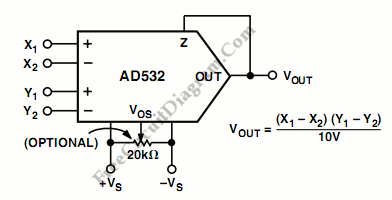The AD532 should be connected as the following figure for operation as a multiplier. We can fed the input differentially to the X and Y inputs, or single ended by simply grounding the unused input. Connect the inputs according to the desired polarity in the output. To close the feedback loop around the op amp, the Z terminal is tied […]

## AD532 Multiplier IC and Its Pin Configurations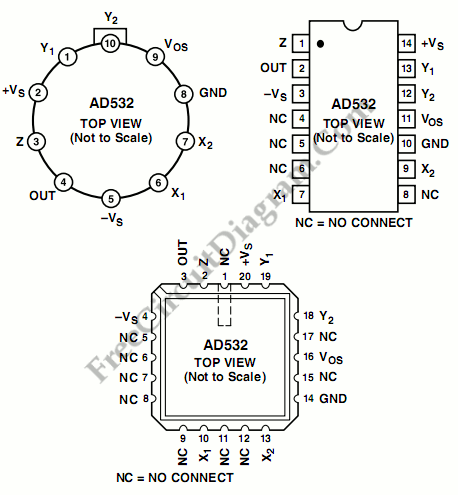The AD532 is the first pre-trimmed single chip monolithic multiplier/divider. This device guarantees a maximum multiplying error of +- 1% and a +-10 V output voltage without the need for any output op amp or external trimming resistors. It’s simplicity of use provides design engineers with an attractive alternative to modular multipliers because the AD532 is internally trimmed. The AD532 […]

## Pulse Width to Analog Voltage Demodulator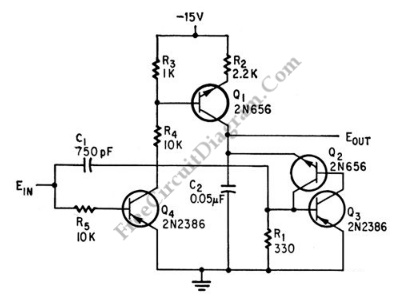This is a schematic diagram of a pulse width to analog demodulator circuit. This circuit is used to demodulate the pulse width to analog voltage level. With input pulse width range of 0 to 1 microsecond, this circuit can produces Output range of 0 to 10 v. The input pulse is integrated and the final value is held until next […]